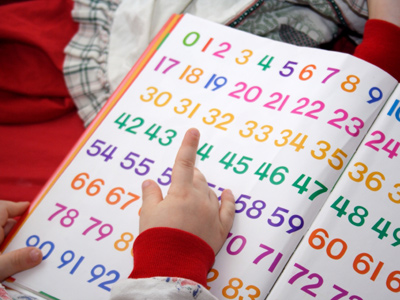Number lines are a useful tool when doing additions or subtractions.

# Year 2 Numbers - Number Lines

This quiz addresses the requirements of the National Curriculum KS1 Maths and Numeracy for children aged 6 and 7 in year 2. Specifically this quiz is aimed at the section dealing with counting on from any given number.

Children in Year 2 should be quite familiar with the number line. They may use it to count on or back, or to calculate additions or subtractions. Understanding the value of numbers means being able to recognise where numbers should come in relation to each other, as well as being able to recognise any missing numbers in a set.

This quiz will help children to use number lines when counting on from any given number.

Question 1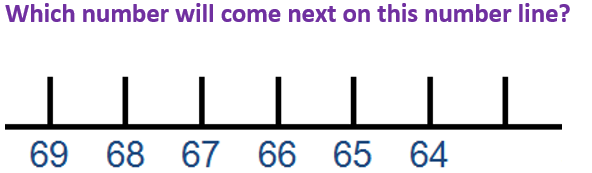60
67
63
70
The numbers are going down, not up
Question 2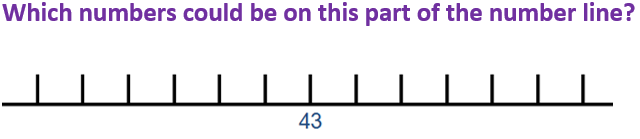39, 44, 47
8, 9, 10
77, 87, 90
20, 23, 27
Counting backwards and forwards from 43 includes the numbers 39, 44 and 47
Question 3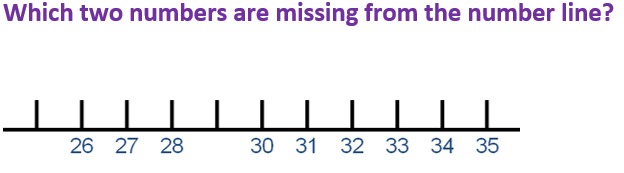26 and 31
25 and 29
29 and 40
23 and 27
25 comes before 26, and 29 comes between 28 and 30
Question 4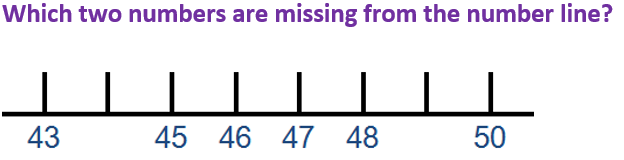44 and 51
45 and 49
51 and 58
44 and 49
Looking at the numbers on either side might be helpful
Question 5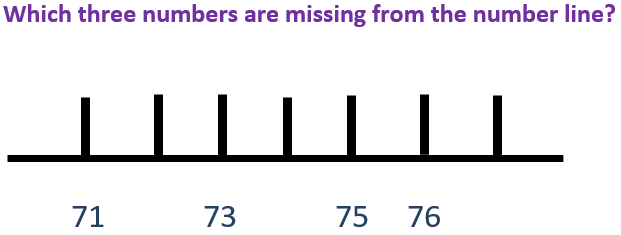75, 76 and 77
72, 74 and 77
74, 75 and 79
80, 90 and 100
Counting up aloud will help identify the missing numbers
Question 6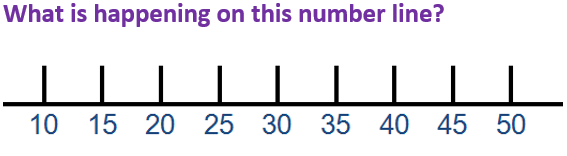The numbers are getting smaller
The numbers are all even
The numbers are going up in 5s
The numbers are all odd
Each number is 5 more than the one before it
Question 7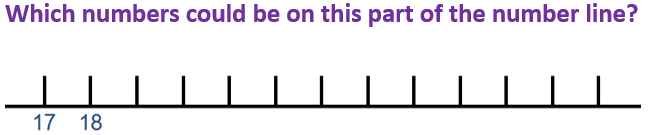88, 89, 90
2, 3, 4
66, 67, 68
20, 21, 22
Counting up from 17 and 18 would include 20, 21 and 22
Question 8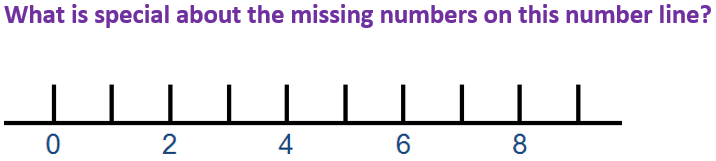They are all even
They are all odd
They are all over 10
They all end in 5
The missing numbers are 1, 3, 5, 7 and 9, which are all odd numbers
Question 9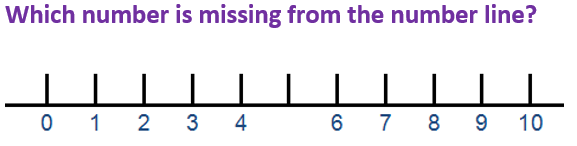5
8
9
2
4 comes before 5, 6 comes after
Question 10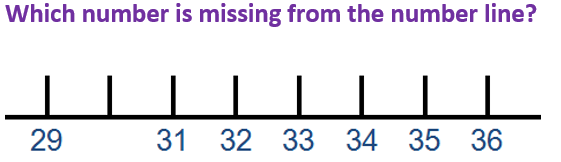25
30
39
28
30 comes before 31, and after 29
You can find more about this topic by visiting BBC Bitesize - What is a number line?

Author:  Angela Smith